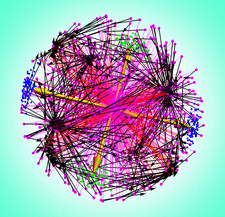# issue contentsFOUNDATIONSADVANCES
ISSN: 2053-2733

# July 2020 issueCover illustration: Finding the unique rotation that best aligns two sets of geometric data is a fundamental problem in many domains, from structural biochemistry and aerospace problems to machine vision and digital heritage applications. Quaternion methods are particularly useful for solving this problem given 3D or 4D data, as shown by A. J. Hanson [Acta Cryst. (2020). A76, 432-457] in this issue. Known methods for aligning 3D data sets such as pairs of matched protein structures are reviewed, and then extended to other domains such as orientation frames: this visualization illustrates the challenging properties of the ambiguous quaternion basins of attraction that appear for unconstrained orientation-frame data.

### The quaternion-based spatial-coordinate and orientation-frame alignment problems

Quaternion methods for obtaining solutions to the problem of finding global rotations that optimally align pairs of corresponding lists of 3D spatial and/or orientation data are critically studied. The existence of multiple literatures and historical contexts is pointed out, and the algebraic solutions of the quaternion approach to the classic 3D spatial problem are emphasized. The treatment is extended to novel quaternion-based solutions to the alignment problems for 4D spatial and orientation data.

## research papers

### Direct recovery of interfacial topography from coherent X-ray reflectivity: model calculations for a 1D interface

The inversion of X-ray reflectivity to reveal the topography of a 1D interface is evaluated through model calculations.

### Extending the Debye scattering equation for diffraction from a cylindrically averaged group of atoms: detecting molecular orientation at an interface

A version of the Debye scattering equation is here developed to calculate diffraction intensity from groups of atoms randomly oriented about an axis, for example when molecules are fixed at an interface in antibody binding. Using an example biomolecule, the high level of sensitivity of the diffraction pattern to the orientation of the molecule and to the direction of the incident beam is shown.

### The chord-length distribution of a polyhedron

The second derivative of the correlation function of any bounded polyhedron has a closed analytic expression that can be determined by the reported procedure.

### Novel phasing method using the origin-free modulus sum function expressed in terms of the absolute electron density

A phasing algorithm based on the origin-free modulus sum function, expressed in terms of absolute electron density instead of the electron density squared, is described and applied to some test cases.

### Spherical-wave X-ray dynamical diffraction Talbot effect inside a crystal

The two-wave dynamical diffraction Talbot effect inside a crystal for the case of spherical-wave illumination of a periodic object is investigated.

### Theoretical study of the properties of X-ray diffraction moiré fringes. III. Theoretical simulation of previous experimental moiré images

Using a recently developed moiré-fringe theory of X-ray diffraction, computer simulations of previous experimental moiré images are presented, for an experimental verification of the moiré-fringe theory and for a theoretical explanation of the peculiar experimental moiré images.

### Derived crystal structure of martensitic materials by solid–solid phase transformation

A mathematical description is provided for the derived lattice/structure for the low-symmetry phase obtained through solid–solid phase transformation. The mathematical concepts are illustrated and verified in a phase-transforming alloy by a synchrotron X-ray diffraction experiment.

### On an extension of Krivovichev's complexity measures

An extension is proposed of the Shannon entropy-based structural complexity measure introduced by Krivovichev, eventually yielding a classification of a crystal structure's complexity into chemical, combinatorial, coordinational, compositional, configurational and crystallographic contributions.